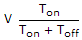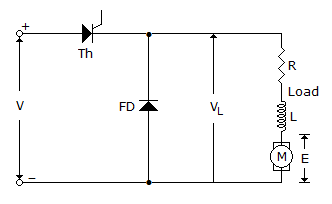# Electronics and Communication Engineering - Power Electronics

### Exercise :: Power Electronics - Section 4

41.

If a step down chopper is fed by a dc voltage V with Ton and Toff are on and off periods thenis

 A. average output voltage B. rms output voltage C. average or rms output voltage depending on load D. neither of the above

Explanation:

No answer description available for this question. Let us discuss.

42.

A voltage VBB is applied across the terminals of a UJT. The emitter voltage at peak point is

 A. hVBB B. (h + 1) VBB C. hVBB + VD D. VD

Explanation:

No answer description available for this question. Let us discuss.

43.

A single phase bridge type cycloconverter uses eight thyristors.

 A. True B. False

Explanation:

No answer description available for this question. Let us discuss.

44.

In the circuit of figure, the waveshape of load current when thyristor conducting isA. constant B. increasing exponentially C. decreasing exponentially D. either (b) or (c)

Explanation:

No answer description available for this question. Let us discuss.

45.

In the basic series inverter circuit, the source supplies load

 A. only in positive half cycle B. both in positive and negative half cycles C. only in part of positive half cycle D. in positive half cycle and part of negative half cycle